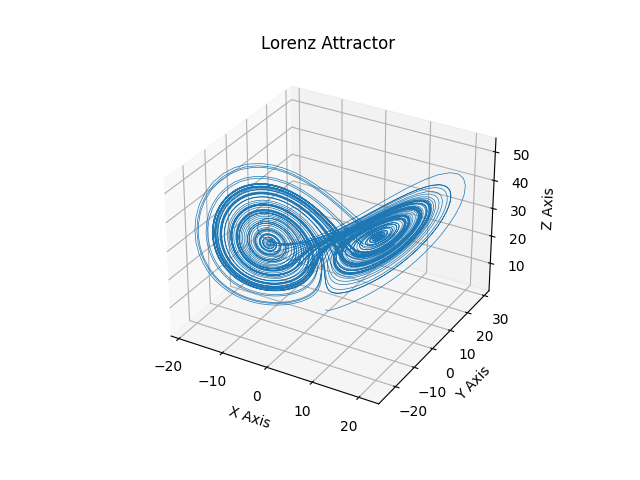# Lorenz Attractor¶

This is an example of plotting Edward Lorenz's 1963 "Deterministic Nonperiodic Flow" in a 3-dimensional space using mplot3d.

Note

Because this is a simple non-linear ODE, it would be more easily done using SciPy's ODE solver, but this approach depends only upon NumPy.import numpy as np
import matplotlib.pyplot as plt

def lorenz(x, y, z, s=10, r=28, b=2.667):
"""
Given:
x, y, z: a point of interest in three dimensional space
s, r, b: parameters defining the lorenz attractor
Returns:
x_dot, y_dot, z_dot: values of the lorenz attractor's partial
derivatives at the point x, y, z
"""
x_dot = s*(y - x)
y_dot = r*x - y - x*z
z_dot = x*y - b*z
return x_dot, y_dot, z_dot

dt = 0.01
num_steps = 10000

# Need one more for the initial values
xs = np.empty(num_steps + 1)
ys = np.empty(num_steps + 1)
zs = np.empty(num_steps + 1)

# Set initial values
xs, ys, zs = (0., 1., 1.05)

# Step through "time", calculating the partial derivatives at the current point
# and using them to estimate the next point
for i in range(num_steps):
x_dot, y_dot, z_dot = lorenz(xs[i], ys[i], zs[i])
xs[i + 1] = xs[i] + (x_dot * dt)
ys[i + 1] = ys[i] + (y_dot * dt)
zs[i + 1] = zs[i] + (z_dot * dt)

# Plot